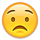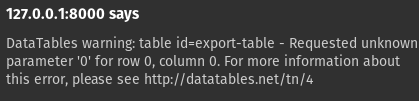# 1 export button for all tables ?

Is this possible ? So that all the datatables can be exported in 1 pdf ?

• ```````\$('table.display').DataTable( {
dom: 'Bfrtip',
buttons: [ {
extend : 'print',
title : title,
},
{
extend: 'pdfHtml5',
title : title,
filename: 'export',
footer: true
}],
language: {
url: url
},
"footerCallback": function ( row, data, start, end, display ) {
var api = this.api(), data;

// Remove the formatting to get integer data for summation
var intVal = function ( i ) {
return typeof i === 'string' ?
i = parseFloat(i.replace(",", ".")):
typeof i === 'number' ?
i : 0;
};
// Total over all pages
total = api
.column( 4 )
.data()
.reduce( function (a, b) {
return intVal(a) + intVal(b);
}, 0 );
pageTotal = api
.column( 4, { page: 'current'} )
.data()
.reduce( function (a, b) {
return intVal(a) + intVal(b);
}, 0 );
// Update footer
pageTotal = pageTotal.toFixed(2);
total = total.toFixed(2);
\$( api.column( 4 ).footer() ).html(
'€'+ total +' total'
);
}
} );
``````

this creates x datatables based on for loops and each with 1 pdf button for each table

Cheers,

Colin

• helped a bit but i am not using the table ID but the class for every table• If you are trying the `\$.merge()` option you should be able to use the `table()` API to individually get each table. See this simple example:
http://live.datatables.net/gihiceju/1/edit

Kevin

• edited May 3

Tried this

``````\$('button').on('click', function() {
var data = ''
var table = \$('.display').DataTable();
for (var i = 0; i < table.length; i++) {
var data = table.table(i).data();
\$.merge( data, table.table(i).data());
}
\$('#export-table').DataTable({
dom: 'Bfrtip',
data: data,
buttons: [{
extend: 'pdfHtml5'
}],
drawCallback: function() {
\$('#export-table_wrapper .buttons-pdf').click()
setTimeout(function() {
\$('#export-table').DataTable().destroy(false);
}, 200)
}
})
})  ;

``````

but my first printout of the table.length is allready 0 for some reason• got a bit further... only issues is my data is not in the in the export for some reason``````\$('button').on('click', function() {
var table = \$('.display').DataTable();
for (var i = 0; i < table.tables().context.length; i++) {
var data = table.table(i).data();
\$.merge( data, table.table(i).data());
}
\$('#export-table').DataTable({
dom: 'Bfrtip',
data: data,
buttons: [{
extend: 'pdfHtml5'
}],
drawCallback: function() {
\$('#export-table_wrapper .buttons-pdf').click()
setTimeout(function() {
\$('#export-table').DataTable().destroy(false);
}, 200)
}
})
})  ;
``````
• You can use the `tables()` API to get all the Datatables or use `table-selector` to limit the Datatables. If you investigate the returned Datatable API you will see there is a `context` property which is an array containing each Datatable. You can use the `length` of that array for your loop.

http://live.datatables.net/gihiceju/2/edit

Kevin

• edited May 3
``````\$('button').on('click', function() {
var table = \$('.display').DataTable();
for (var i = 0; i < table.tables().context.length; i++) {
var data = table.table(i).context;
\$.merge( data, table.table(i).context );
}
\$('#export-table').DataTable({
dom: 'Bfrtip',
data: data,
buttons: [{
extend: 'pdfHtml5'
}],
drawCallback: function() {
\$('#export-table_wrapper .buttons-pdf').click()
setTimeout(function() {
\$('#export-table').DataTable().destroy(false);
}, 200)
}
})
})  ;
``````

almost theire! Now Only this error left
when alerting data it says 2 times an object.• ``````\$('button').on('click', function() {
var table = \$('.display').DataTable();
for (var i = 1; i < table.tables().context.length; i++) {
var data = table.table(i).data();
\$.merge( data, table.table(i).data());
}
\$('#export-table').DataTable({
dom: 'Bfrtip',
data: data,
buttons: [{
extend: 'pdfHtml5'
}],
drawCallback: function() {
\$('#export-table_wrapper .buttons-pdf').click()
setTimeout(function() {
\$('#export-table').DataTable().destroy(false);
}, 200)
}
})
})  ;

``````

Also tried this one...still nothing in the export except for the headers.

• Maybe you need to do something like this:

``````            var table = \$('.display').DataTable();
var data = [];
for (var i = 1; i < table.tables().context.length; i++) {
\$.merge( data, table.table(i).data());
}
``````

If this doesn't help please post a test case example so we can help debug.

Kevin

• now it works but it prints on 1 table but the data for each table is not seperatedhttp://live.datatables.net/gihiceju/8/edit?html,js,console,output

• so the title is an H5 automatic generated

what i want in the pdf is:

H5 as title of the Datatable
datatable1
H5 as title of datatable2
Datatable2

or is this not a good way ?

• i saw theire was a mistake in the url docs

http://live.datatables.net/gihiceju/10/

On that last URL there's a console error - `data` is undefined. I changed it to be `data_export` and it's working nicely : http://live.datatables.net/gihiceju/11/edit

Colin

• edited May 4

thanks ! not just 100% though. if you could help me with it ?

When the first/last name changes. thats a new datatable. but i would want a title between every export.
Is that something that would work ? so:
title - datatable1 (where title is an h5 on my HTML )
title - datatable2

or can you "name" your datatable ?

• Also the footer is not on the table